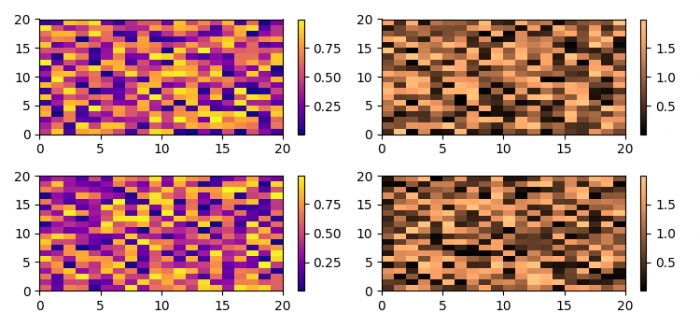# How to plot a pcolor colorbar in a different subplot in Matplotlib?

To plot a pcolor colorbar in a different subplot in Matplotlib, we can take the following steps −

• Set the figure size and adjust the padding between and around the subplots.
• Create a figure and a set of subplots wuth two rows and two columns.
• Make a list of colormaps.
• Iterate the axes and create a pseudocolor plot with a non-regular rectangular grid.
• Make colorbars with the same axes of pcolormesh.
• To display the figure, use show() method.

## Example

import matplotlib.pyplot as plt
import numpy as np

plt.rcParams["figure.figsize"] = [7.50, 3.50]
plt.rcParams["figure.autolayout"] = True

fig, axs = plt.subplots(2, 2)
cm = ['plasma', 'copper']

for col in range(2):
for row in range(2):
ax = axs[row, col]
pcm = ax.pcolormesh(np.random.random((20, 20)) * (col + 1), cmap=cm[col])
fig.colorbar(pcm, ax=ax)

plt.show()

## Output